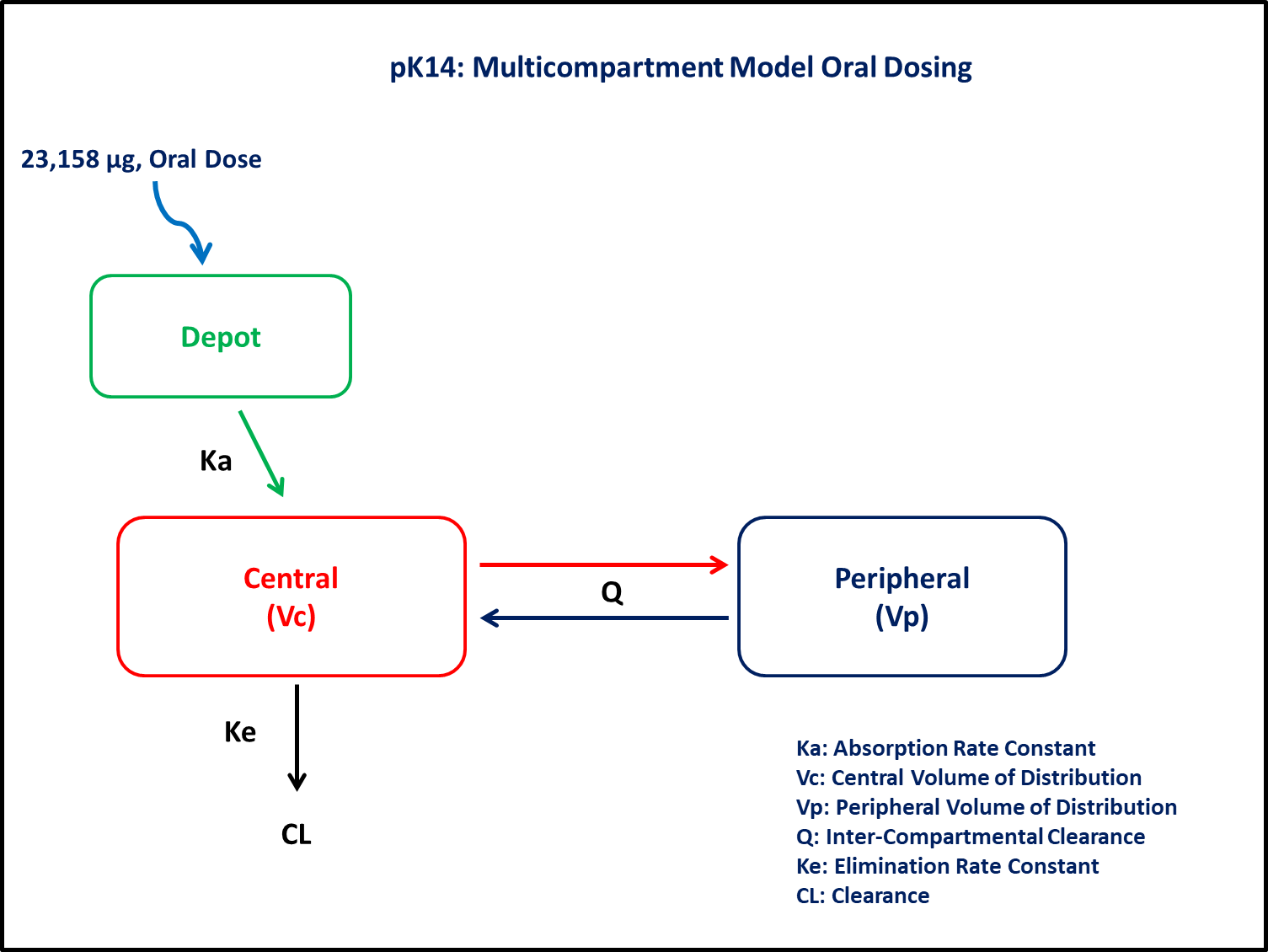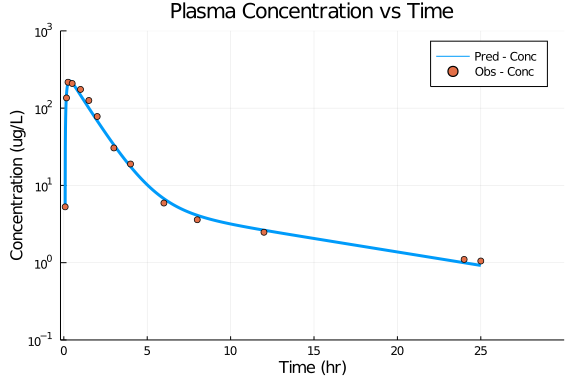# Exercise PK14 - Multi-compartment oral data

### Background

• Structural model - Two compartment linear elimination and first order absorption with lagtime

• Route of administration - Oral

• Dosage regimens - 23,158 μg single dose

• Subject - 1### Learning Outcome

In this model, fitting of po data will help you to understand the disposition of drug following lag time in absorption

### Objectives

In this tutorial, you will learn how to build two compartment model with lagtime in oral absorption and to simulate the model for a single subject.

### Libraries

call the "necessary" libraries to get start.

using Pumas
using Plots
using CSV
using StatsPlots
using Random


### Model

In this two compartment model, we administer dose to Depot and Central compartment.

pk_14           = @model begin
@param begin
tvka        ∈ RealDomain(lower=0)
tvvc        ∈ RealDomain(lower=0)
tvvp        ∈ RealDomain(lower=0)
tvcl        ∈ RealDomain(lower=0)
tvq         ∈ RealDomain(lower=0)
tvlag       ∈ RealDomain(lower=0)
Ω           ∈ PDiagDomain(4)
σ²_prop     ∈ RealDomain(lower=0)
end

@random begin
η           ~ MvNormal(Ω)
end

@pre begin
Ka          = tvka * exp(η)
Vc          = tvvc * exp(η)
Vp          = tvvp * exp(η)
Cl          = tvcl * exp(η)
Q           = tvq
lags        = (Depot = tvlag,)
end

@dynamics begin
Depot'      = -Ka*Depot
Central'    =  Ka*Depot - (Cl/Vc)*Central + (Q/Vp)*Peripheral - (Q/Vc)*Central
Peripheral' =  (Q/Vc)*Central - (Q/Vp)*Peripheral
end

@derived begin
cp          = @. Central/Vc
dv          ~ @. Normal(cp, sqrt(cp^2*σ²_prop))
end
end

PumasModel
Parameters: tvka, tvvc, tvvp, tvcl, tvq, tvlag, Ω, σ²_prop
Random effects: η
Covariates:
Dynamical variables: Depot, Central, Peripheral
Derived: cp, dv
Observed: cp, dv


### Parameters

Parameters provided for simulation. tv represents the typical value for parameters.

• Ka - Absorption rate constant (hr⁻¹)

• Vc - Volume of central compartment (L)

• Vp - Volume of pepheral Compartmental (L)

• Cl - Clearance (L/hr)

• Q - Intercompartmental clearance (L/hr)

• lag - Absorption lagtime (hr)

param = ( tvka    = 10,
tvvc    = 82.95,
tvcl    = 54.87,
tvq     = 10.55,
tvlag   = 0.078,
tvvp    = 107.9,
Ω       = Diagonal([0.0,0.0,0.0,0.0]),
σ²_prop = 0.0125)

(tvka = 10, tvvc = 82.95, tvcl = 54.87, tvq = 10.55, tvlag = 0.078, tvvp =
107.9, Ω = [0.0 0.0 0.0 0.0; 0.0 0.0 0.0 0.0; 0.0 0.0 0.0 0.0; 0.0 0.0 0.0
0.0], σ²_prop = 0.0125)


### Dosage Regimen

Single Subject receives an oral dose of 23158 μg at time=0

ev1  = DosageRegimen(23158, time=0, cmt=1)
sub1 = Subject(id=1, events=ev1)

Subject
ID: 1
Events: 1


### Simulation

Lets simulate plasma concentration after oral dosing.

Random.seed!(123)
sim_sub1 = simobs(pk_14,sub1,param,obstimes=0.08:0.01:25)


### Dataframe & Plot

Convert to a DataFrame for plotting.

df1    = DataFrame(sim_sub1)
df1_dv = filter(x -> x.time in [0.08,0.16,0.25,0.5,1,1.5,2,3,4,6,8,12,24,25], df1)

@df df1 plot(:time, :cp, yaxis = :log,
label="Pred - Conc", xlabel="Time (hr)", ylabel="Concentration (ug/L)",
title="Plasma Concentration vs Time", linewidth=3,
xticks=[0,5,10,15,20,25], yticks=[0.1,1,10,100,1000], ylims=(0.1,1000), xlims=(-0.2,30))
@df df1_dv scatter!(:time, :dv, label="Obs - Conc")